# Catch up on the latest AI articles# What Causes Catastrophic Forgetting?

3 main points
✔️ Research on the mechanism of catastrophic forgetting
✔️ Discovered that deep layers of neural networks affect catastrophic forgetting
✔️ Discovered the relationship between the similarity between tasks and catastrophic forgetting

Anatomy of Catastrophic Forgetting: Hidden Representations and Task Semantics
written by Vinay V. RamaseshEthan DyerMaithra Raghu
(Submitted on 14 Jul 2020)
Comments: Accepted to ICLR2021.

Subjects: Machine Learning (cs.LG); Computer Vision and Pattern Recognition (cs.CV); Machine Learning (stat.ML)

## First of all

Catastrophic forgetting, which is a significant degradation in performance on existing tasks when a trained model is further trained, is a major challenge in deep learning models.

In the paper presented in this article, we investigate the representation of neural networks and various methods for suppressing catastrophic forgetting, and find that catastrophic forgetting is caused in the layer close to the output. We also investigate the relationship between the similarity between multiple tasks performed and catastrophic forgetting.

## Experiment

As an investigation of catastrophic forgetting, we conduct experiments using a variety of task-model architectures.

### task

The following tasks are used in the experiment. Since multiple tasks are learned sequentially in the experiment, each task consists of several (two) tasks.

• Split CIFAR-10: We use a 10-class dataset split into two tasks with 5 classes each.
• Input distribution shift CIFAR-100: The task is to distinguish the upper classes of CIFAR-100, but the input data is a different set of distributions within the same upper class, resulting in a distribution shift.
• CelebA Attribute Prediction: predicts that the input data for the two tasks is either male or female, and either a smile or an open mouth.
• ImageNet upper class prediction: This is the same task as CIFAR100 described above.

### model

Three common architectures used for image classification tasks are utilized, as follows

An example of catastrophic forgetting when these models are trained on two tasks in sequence is shown below.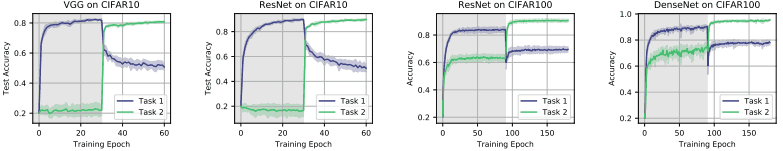Among the aforementioned tasks, the split CIFAR10/input distribution shift CIFAR-100 has been used in the experiments.

When task 2 is trained after task 1 is completed, we can see that the performance of task 1 is significantly degraded.

### Catastrophic forgetting and the representation of hidden layers

To begin, we investigate the relationship between feature representation in the hidden layer within a neural network and catastrophic forgetting. It aims to answer the following questions.

• Do all parameters and layers in the network cause catastrophic forgetting as well?
• Are specific parameters or layers in the network the primary drivers of catastrophic forgetting?

As a result of a series of experiments described below, we found that the cause of catastrophic forgetting is in the layer closest to the output (the upper layer).

Freezing of specific layers

In order to investigate the effect of a specific layer in the network on forgetting, we conduct an experiment to freeze the weights of a specific layer (freeze: fix the parameters without updating). Specifically, after training in task 1, we freeze the layers from the lowest layer (the layer closest to the input) to a specific layer, and train only the remaining layers in task 2.

The results of the experiment are as follows.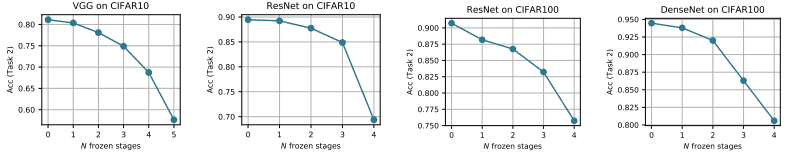The larger the number on the horizontal axis, the more the weights are frozen until the layer closer to the output (upper layer). As can be seen from this figure, the accuracy for task 2 does not decrease much even if the weights of the lower layers are fixed.

Therefore, we expect that the feature representations in the lower layer can be reused without updating between tasks 1 and 2, and that the upper layer is the main source of catastrophic forgetting.

If the layer is not frozen

As mentioned earlier, the feature representation of the lower layer can be reused between tasks 1 and 2 (even if the feature representation of the lower layer is used as it is, good performance can be achieved only by updating the upper layer).

Then, does the feature representation of the lower layer change significantly even if the normal training is performed without freezing the layer weights? To investigate this, we use Centered Kernel Alignment (CKA ), which is a method to measure the similarity of neural network representations. CKA shows how similar the representations of two layers are by a scalar value from 0 to 1.

Specifically, for a layer activation matrix $X \in R^{n×p},Y \in R^{n×p}$consisting of ($n$) data points and ($p$) neurons, CKA is obtained as follows

$CKA(X,Y)=\frac{HSIC(XX^T, YY^T)}{\sqrt{HSIC(XX^T, XX^T)}\sqrt{HSIC(YY^T, YY^T)}}$

HSIC stands for Hilbert-Schmidt Independence Criterion.

The following figure shows the similarity between the layer representations calculated by CKA before and after learning task 2.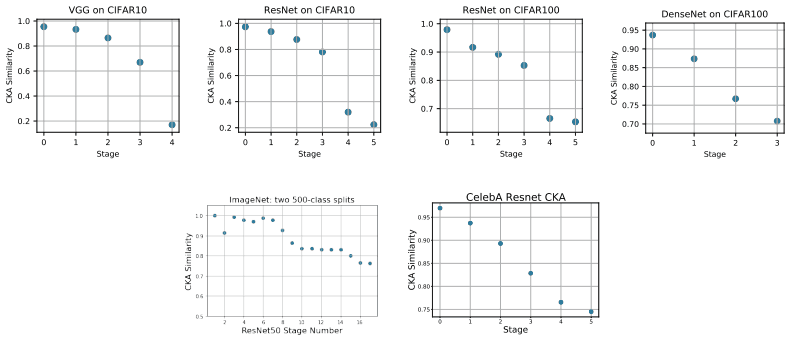As shown in the figure, the similarity of the lower layers before and after learning task 2 is high, while the similarity of the upper layers is significantly lower.

Resetting the layer

We also conduct additional experiments to reveal that the upper layers have a significant effect on catastrophic forgetting. Specifically, after sequentially learning tasks 1 and 2, we rewind $N$ successive layers from the bottom or top layer to the state before learning task 2 (just after the completion of learning task 1).

The result of this is shown in the following figure.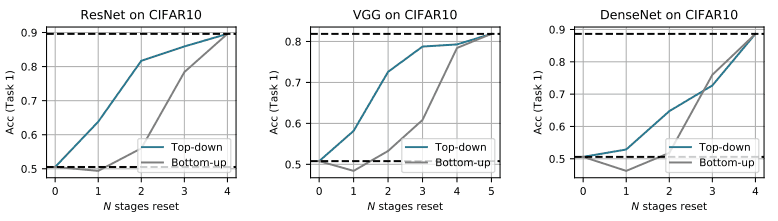Top-down shows the performance when resetting N layers from the top layer (near the output), and bottom-up shows the performance when resetting N layers from the bottom layer. In general, resetting the layers near the top gives higher performance than resetting the layers near the bottom.

This indicates that the upper layers have a strong influence on catastrophic forgetting.

Reuse of feature representation and elimination of subspace

The change in representation during sequential learning of the task is further investigated by analyzing subspace similarity.

The subspace similarity, $X \in R^{n×p}$, is calculated by PCA (Principal Component Analysis) using the matrix $V_k$ with columns from the first to the $k$ principal components, and $U_k$ obtained in the same way for $Y\in R^{n×p}$, as follows.

$SubspaceSim_k(X,Y)=\frac{1}{k}||V^T_kU_k||^2_F$

Here, the subspace similarity is calculated for each of the following three conditions.

• (1) X: model trained on task 1, Y: model trained on task 2
• (2) X: Model trained on task 1, Y: Model trained sequentially on task 1 and then task 2.
• (3) X: Model trained on task 2, Y: Model trained sequentially on task 1 and then task 2.

The subspace similarity in this case is shown in the following figure.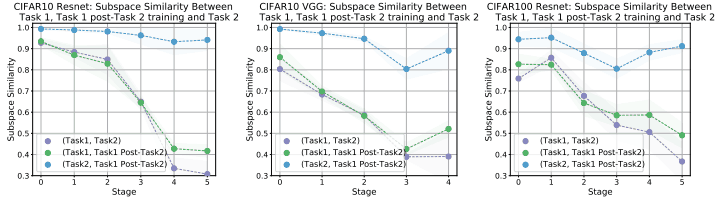As can be seen from the comparison between before and after learning task 2 (2: shown in green), the similarity of the upper layers is significantly reduced before and after learning task 2, indicating a significant change in the subspace.

As in the analysis using CKA, we can see that the lower-level representations do not change much between Tasks 1 and 2, but the upper-level representations change significantly.

Forgetting mitigation methods and feature representation

The experiments so far have shown that catastrophic forgetting is mainly caused by the influence of the upper layer.

So, how does the upper layer change when we use a method that prevents catastrophic forgetting (continuous learning)?

In fact, we show below the subspace similarity when using methods such as Replay Buffer, EWC (Elastic Weight Consolidation) and SI (Synaptic Intelligence ).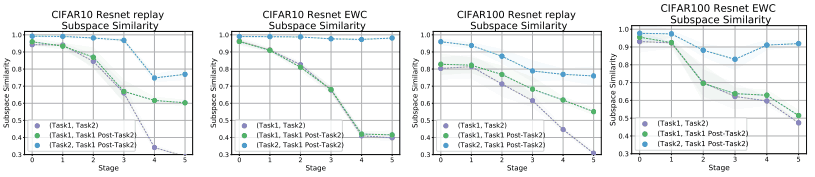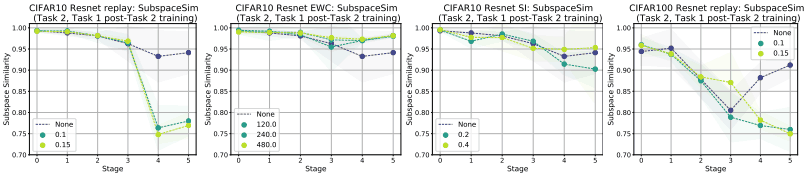The upper panel shows the case with various relaxation methods, and the lower panel shows the case with varying the intensity of the relaxation methods (Task2, Task1 Post-Task2).

Since the replay method uses the data from Task 1 when training Task 2, the similarity in the upper layer of (Task2, Task1 Post-Task2) decreases, while the similarity in EWC/SI remains high. This may suggest that EWC/SC encourages the reuse of feature representations, whereas the replay method uses orthogonal subspaces.

The CKA values are also compared as follows.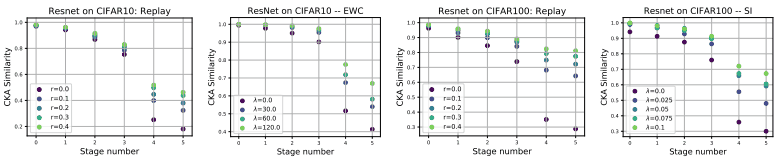In this figure, the CKA values are shown for the three previously mentioned relaxation methods applied at different intensities ($r$ or $\lambda$).

As can be seen from the figure, the stronger the relaxation technique is applied, the more the similarity improves, especially in the upper layers (the change in the upper layers is suppressed).

However, it is an open question whether the improvement in similarity in the upper layer is due to the reuse of feature representations in the upper layer, or because the representations of tasks 1 and 2 are stored in orthogonal subspaces, respectively.

Relationship between inter-task similarity and catastrophic forgetting

In our previous experiments, we investigated the relationship between catastrophic forgetting and feature representation in the network. In subsequent experiments, we will investigate the effect of the relationship between successive tasks on catastrophic forgetting.

It aims to answer questions such as.

• Does a higher degree of similarity between tasks reduce catastrophic forgetting?

To answer this question, we experimented with the relationship between similarity between tasks and catastrophic forgetting, and the results are shown below.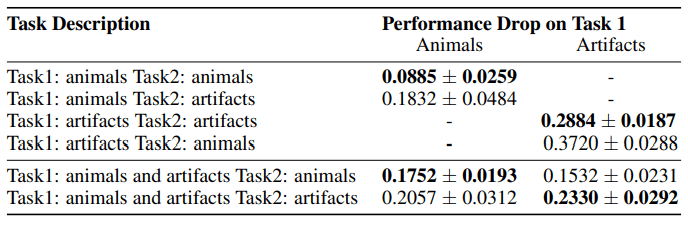In this table, we show the performance degradation when Task1 and Task2 are composed of artifacts and animal classes using ImageNet.

For example, in Task1: animals Task2: animals, after learning the 10 animal image classification tasks (Task1), it executes 10 different animal image classification tasks (Task2).

If we assume that the similarity between tasks mitigates catastrophic forgetting, we should expect that the performance degradation should be less when two tasks are similar (animals-animals or artifacts-artifacts). However, in reality, the performance degradation is much worse for Task1:artifacts Task2:artifacts, which is contrary to the above assumption. Similarly, the learning curves for the experiments with tasks constructed based on objects or animals are shown below.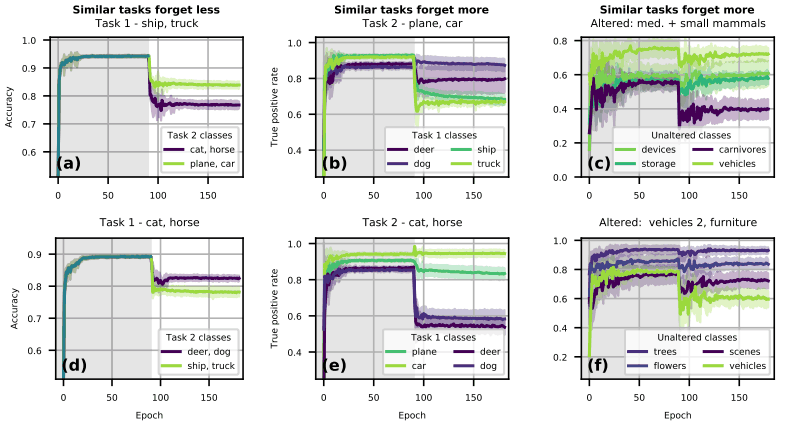For example, in (a) of the figure, Task 1 contains the ship and truck classes. In this case, when we experiment with two types of Task 2 (cat,horse or plane,car), the performance is higher when we train on the same object as Task 1 (plane,car).

In other words, catastrophic forgetting is weakened when similar tasks are learned. This is a similar result in (d).

On the other hand, in (b), when two classes of objects and animals (ship, truck, deer, dog) are trained in Task 1, and then objects (plane, car) are trained, the accuracy for ship and truck, which are also objects, deteriorates.

In other words, the catastrophic forgetting is rather severe due to the learning of similar tasks. This is the same result in (e).

The same is true for (c,f), where the experiments were performed on CIFAR-100, and the performance on the class with a changed input distribution (Altered) and the similar class (no shift in distribution: Unaltered) is degraded. Thus, we found that when tasks are similar, there are times when performance improves and times when it decreases.

So what is the relationship between inter-task similarity and catastrophic forgetting? Without going into the details of the process, the paper defined and tested a measure of similarity between tasks, and showed that catastrophic forgetting is strongest when the similarity is moderate.

This is shown in the figure below.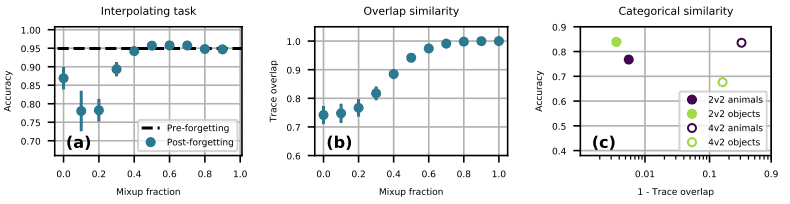In Fig. (a), we first train the binary classification task, and then show the accuracy when training on a dataset that is a mixture of other tasks and the dataset at a fixed fraction (mixup fraction).
As shown in the figure, there is a particular performance degradation when the mixup fraction is between 0.1 and 0.2.
Figure (b) shows the task similarity (trace overlap) for the mixing ratio of the two data sets, and shows that the task similarity varies monotonically with the mixing ratio.
Figure (c) shows the similarity between Task 1 and 2 in the aforementioned tasks (consisting of a to f). Here, ● corresponds to (a) in the figure and ○ to (b) in the figure.

The actual result is that yellow-green performs lower in the yellow-green/purple ● and yellow-green in the yellow-green/purple ○ than the other color.

The reason for this can be explained as follows.

• When compared with yellow-green and purple ●, the accuracy is lower in purple, where the similarity between tasks is lower
• When compared with the yellow-green and purple circles, the accuracy of the yellow-green circle is lower because the similarity of the yellow-green circle is medium.

Thus, based on the finding that catastrophic forgetting is most reduced when task similarity is moderate, we can explain the results for the task (consisting of a-f) described earlier.

## Summary

In the paper introduced in this article, the mechanism of catastrophic forgetting, the representation of neural networks, continuous learning methods, and the relationship with tasks were investigated, and the following two major findings were obtained.

• Catastrophic forgetting is largely contributed by the upper layer (the layer close to the output).
• Catastrophic forgetting is strongest when inter-task similarity is moderate (based on the measures defined in the paper).

Although these results do not provide a direct solution to catastrophic forgetting, they do provide very meaningful wisdom for research on methods to deter catastrophic forgetting.

If you have any suggestions for improvement of the content of the article,
please contact the AI-SCHOLAR editorial team through the contact form.